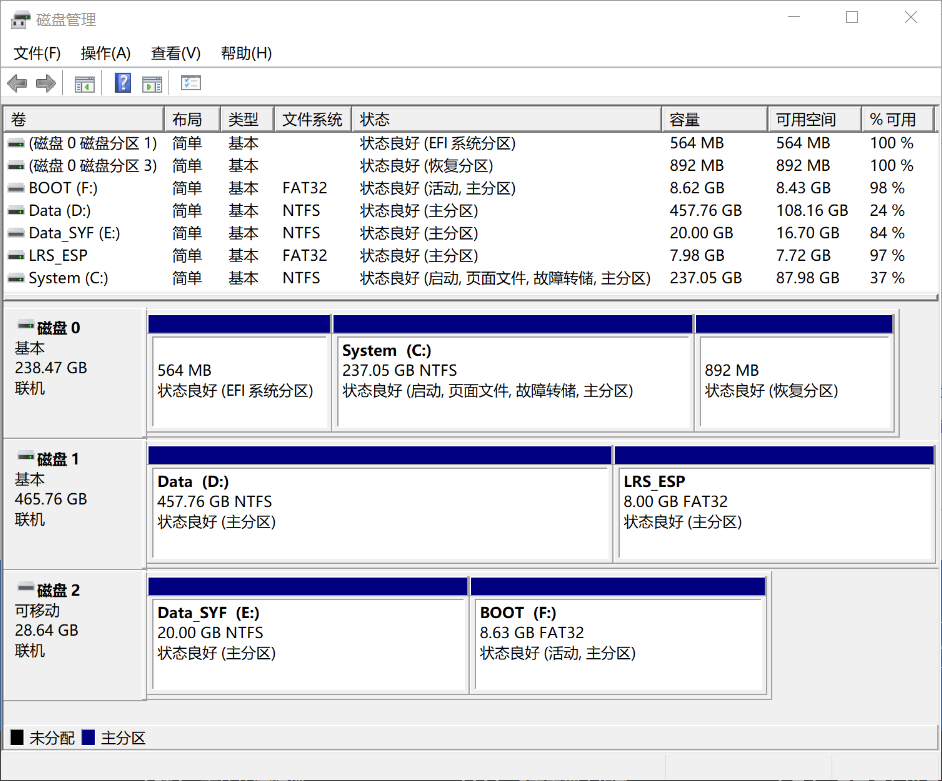## 我的磁盘这样分区

Windows 10 创意者更新之后,终于修复了 "U盘只显示第一个分区" 的 BUG, 此时本机的固态、机械、U盘分区情况就非常清爽舒服了.- 阅读剩余部分 -

# 原文

Farmer John completed his new barn just last week, complete with all the latest milking technology. Unfortunately, due to engineering problems, all the stalls in the new barn are different. For the first week, Farmer John randomly assigned cows to stalls, but it quickly became clear that any given cow was only willing to produce milk in certain stalls. For the last week, Farmer John has been collecting data on which cows are willing to produce milk in which stalls. A stall may be only assigned to one cow, and, of course, a cow may be only assigned to one stall.
Given the preferences of the cows, compute the maximum number of milk-producing assignments of cows to stalls that is possible.

The input includes several cases. For each case, the first line contains two integers, N (0 <= N <= 200) and M (0 <= M <= 200). N is the number of cows that Farmer John has and M is the number of stalls in the new barn. Each of the following N lines corresponds to a single cow. The first integer (Si) on the line is the number of stalls that the cow is willing to produce milk in (0 <= Si <= M). The subsequent Si integers on that line are the stalls in which that cow is willing to produce milk. The stall numbers will be integers in the range (1..M), and no stall will be listed twice for a given cow.

For each case, output a single line with a single integer, the maximum number of milk-producing stall assignments that can be made.

- 阅读剩余部分 -

## 素数筛法

2017-04-16更新:

void init_prime(int n) //欧拉素数筛法
{
for(int i = 2; i <= n; i++)
{
if(!prime[i])
prime[++prime] = i;
for(int j = 1; j <= prime && prime[j] <= n / i; j++)
{
prime[prime[j] * i] = 1;
if(i % prime[j] == 0)
break;
}
}
}

- 阅读剩余部分 -

## 快速幂

int ksm(int a, int b, int n)
{
a %= n;
int ans = 1;
while(b)
{
if(b%2 == 1)
ans = ans*a%n;
b /= 2;
a = a*a%n;
}
return ans;
}

5
50 43 1 58 60
87 22 5 62 71
62 98 97 27 38
56 57 96 73 71
92 36 43 27 95

144

- 阅读剩余部分 -